# 5 58 A 2 5 L flask at

• Slides: 13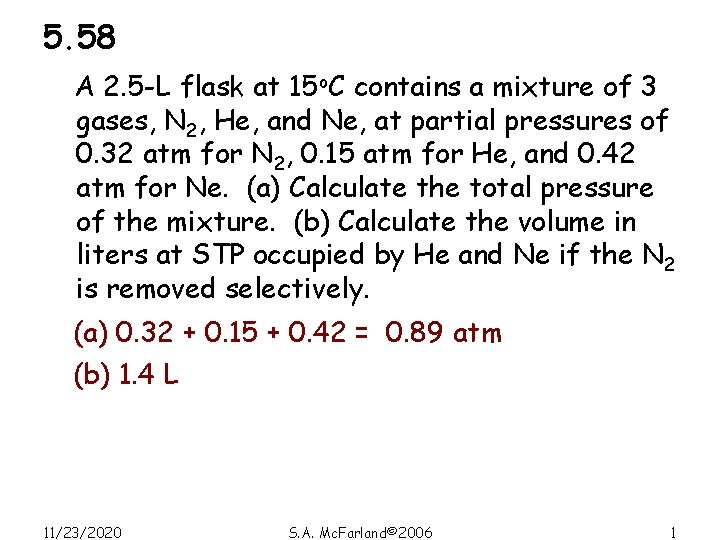5. 58 A 2. 5 -L flask at 15 o. C contains a mixture of 3 gases, N 2, He, and Ne, at partial pressures of 0. 32 atm for N 2, 0. 15 atm for He, and 0. 42 atm for Ne. (a) Calculate the total pressure of the mixture. (b) Calculate the volume in liters at STP occupied by He and Ne if the N 2 is removed selectively. (a) 0. 32 + 0. 15 + 0. 42 = 0. 89 atm (b) 1. 4 L 11/23/2020 S. A. Mc. Farland© 2006 1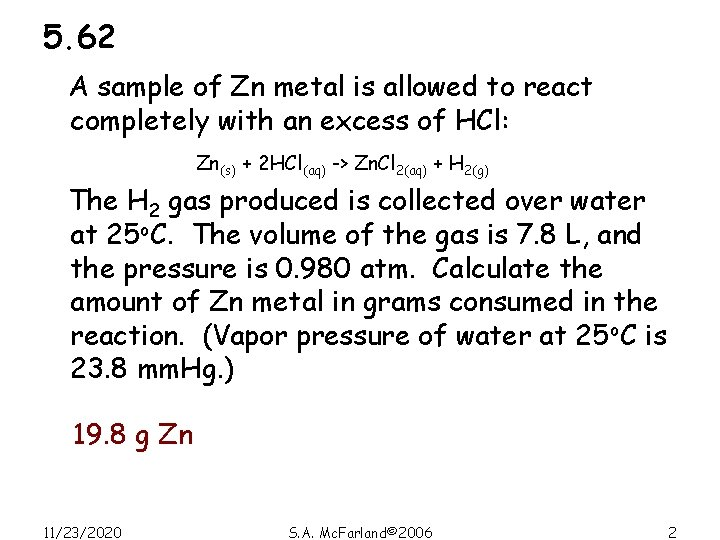5. 62 A sample of Zn metal is allowed to react completely with an excess of HCl: Zn(s) + 2 HCl(aq) -> Zn. Cl 2(aq) + H 2(g) The H 2 gas produced is collected over water at 25 o. C. The volume of the gas is 7. 8 L, and the pressure is 0. 980 atm. Calculate the amount of Zn metal in grams consumed in the reaction. (Vapor pressure of water at 25 o. C is 23. 8 mm. Hg. ) 19. 8 g Zn 11/23/2020 S. A. Mc. Farland© 2006 25. 64 A sample of ammonia (NH 3) gas is completely decomposed to nitrogen and hydrogen gases over heated iron wool. If the total pressure is 866 mm. Hg, calculate the partial pressures of N 2 and H 2. 2 NH 3 -> N 2 + 3 H 2 1 mol N 2: 3 mol H 2 so XN 2 is 0. 25 and XH 2 is 0. 75 PN 2 = XN 2 x PT = 217 mm. Hg PH 2 = XH 2 x PT = 650 mm. Hg 11/23/2020 S. A. Mc. Farland© 2006 3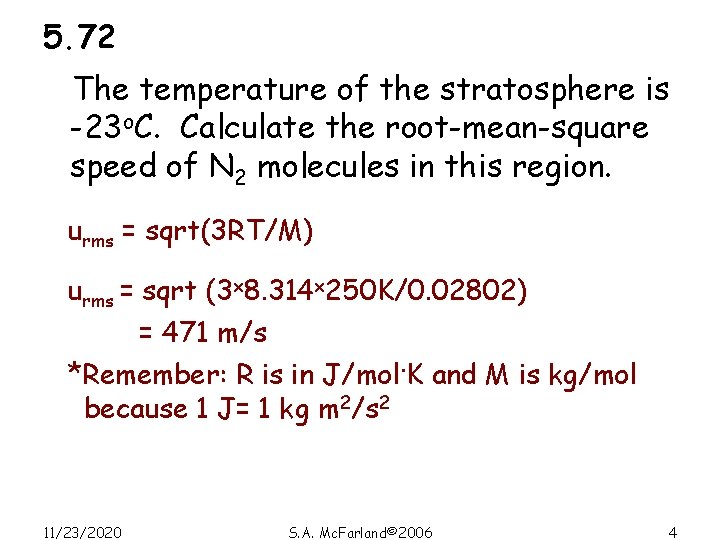5. 72 The temperature of the stratosphere is -23 o. C. Calculate the root-mean-square speed of N 2 molecules in this region. urms = sqrt(3 RT/M) urms = sqrt (3 x 8. 314 x 250 K/0. 02802) = 471 m/s *Remember: R is in J/mol. K and M is kg/mol because 1 J= 1 kg m 2/s 2 11/23/2020 S. A. Mc. Farland© 2006 4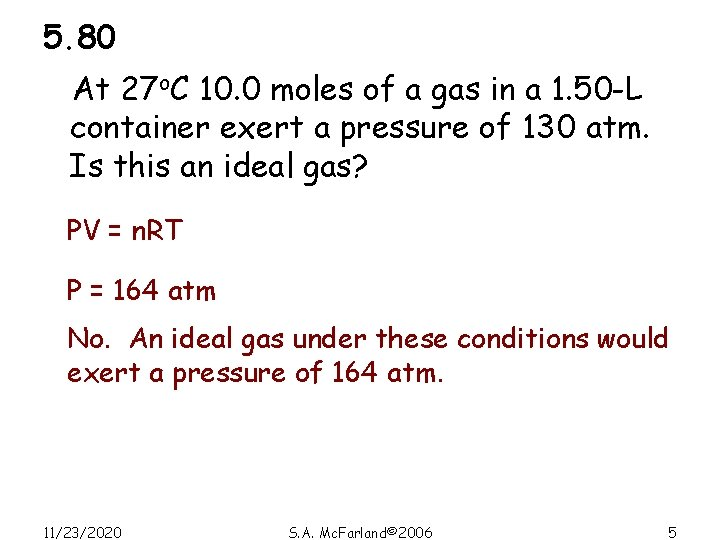5. 80 At 27 o. C 10. 0 moles of a gas in a 1. 50 -L container exert a pressure of 130 atm. Is this an ideal gas? PV = n. RT P = 164 atm No. An ideal gas under these conditions would exert a pressure of 164 atm. 11/23/2020 S. A. Mc. Farland© 2006 5Energy relationships in chemical reactions Chapter 6Energy is the capacity to do work • Radiant energy is earth’s primary energy source and comes from the sun • Thermal energy is the energy associated with the random motion of atoms and molecules • Chemical energy is the energy stored within the bonds of chemical substances • Nuclear energy is the energy stored within the collection of neutrons and protons in the atom • Electrical energy is the energy associated with the flow of electrons • Potential energy is the energy available by virtue of an object’s position 11/23/2020 S. A. Mc. Farland© 2006 7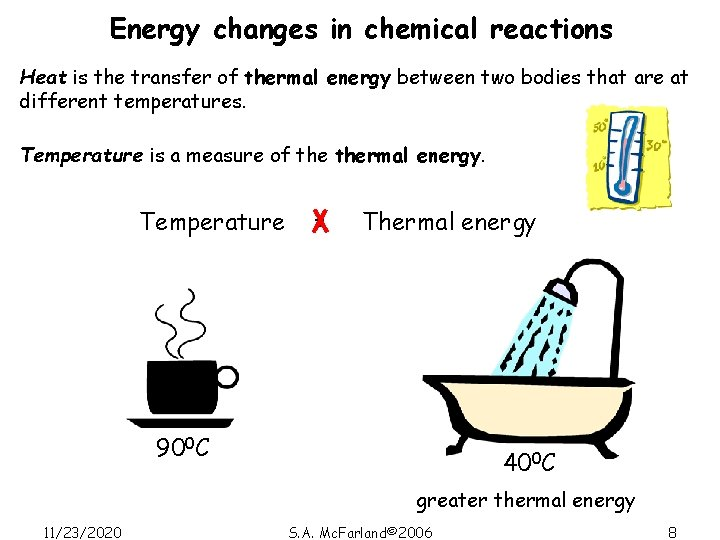Energy changes in chemical reactions Heat is the transfer of thermal energy between two bodies that are at different temperatures. Temperature is a measure of thermal energy. Temperature = Thermal energy 900 C 400 C greater thermal energy 11/23/2020 S. A. Mc. Farland© 2006 8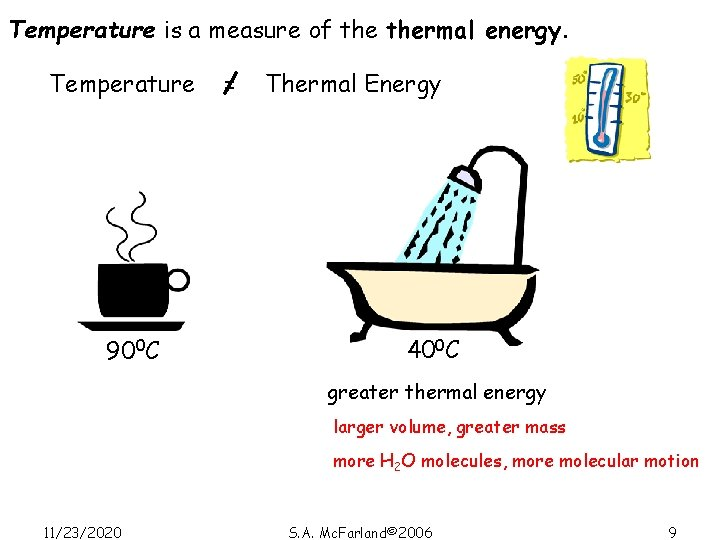Temperature is a measure of thermal energy. Temperature 900 C = Thermal Energy 400 C greater thermal energy larger volume, greater mass more H 2 O molecules, more molecular motion 11/23/2020 S. A. Mc. Farland© 2006 9Thermochemistry is the study of heat change in chemical reactions. The system is the specific part of the universe that is of interest in the study. SURROUNDINGS SYSTEM open Exchange: mass & energy 11/23/2020 closed isolated energy nothing S. A. Mc. Farland© 2006 10Exothermic process is any process that gives off heat – transfers thermal energy from the system to the surroundings. 2 H 2 (g) + O 2 (g) 2 H 2 O (l) + energy H 2 O (g) H 2 O (l) + energy Endothermic process is any process in which heat has to be supplied to the system from the surroundings. energy + 2 Hg. O (s) energy + H 2 O (s) 11/23/2020 2 Hg (l) + O 2 (g) S. A. Mc. Farland© 2006 H 2 O (l) 11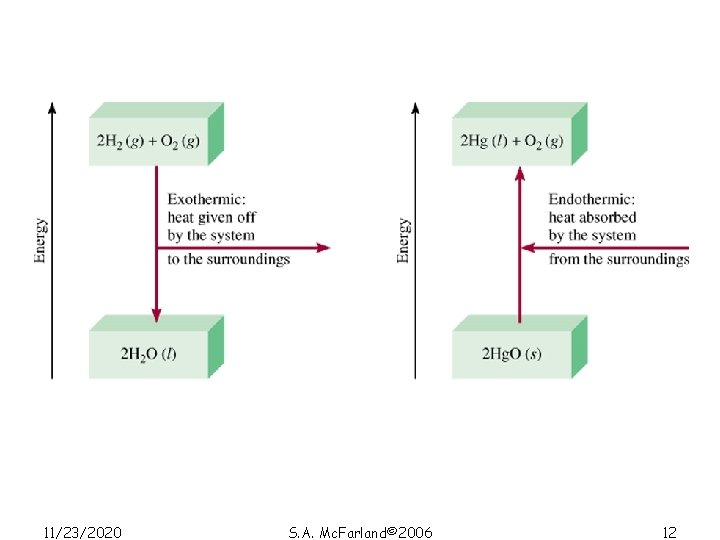11/23/2020 S. A. Mc. Farland© 2006 12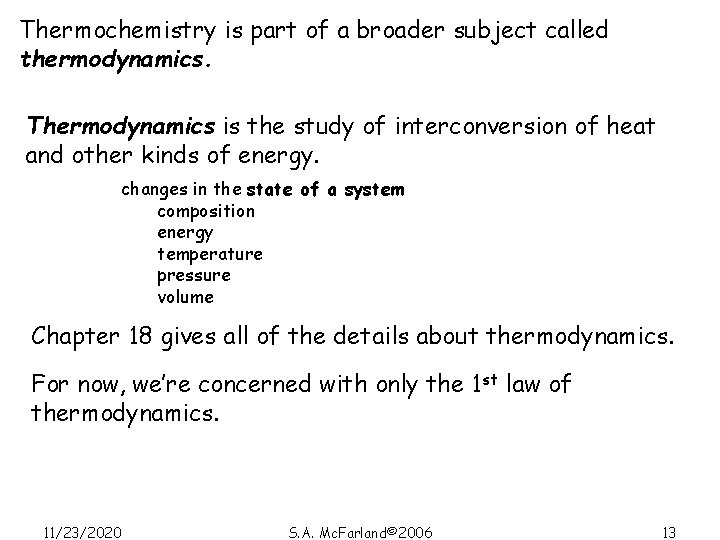Thermochemistry is part of a broader subject called thermodynamics. Thermodynamics is the study of interconversion of heat and other kinds of energy. changes in the state of a system composition energy temperature pressure volume Chapter 18 gives all of the details about thermodynamics. For now, we’re concerned with only the 1 st law of thermodynamics. 11/23/2020 S. A. Mc. Farland© 2006 13